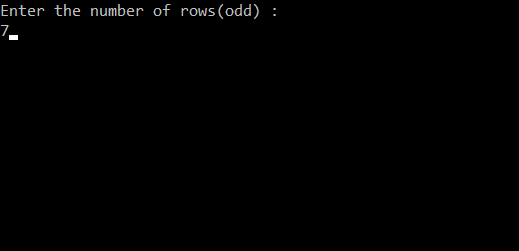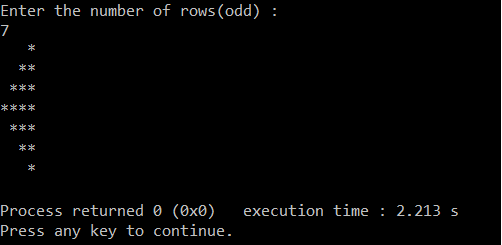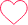# Print Inverted Half Diamond Star Pattern

*

**

***

****

***

**

*

## PREREQUISITE:

Basic knowledge of C language and use of loops.

## ALGORITHM:

1. Take the number of rows as input from the user and store it in any variable.(‘r‘ in this case).
2. Run a loop ‘r’ number of times to iterate through each of the rows. From i=0 to i<r. The loop should be structured as for( i=0 ; i<r : i++).
3. Use an if condition to to print the top half of the diamond. if (i<=r/2). Then run a loop from k=r/2 to k>i. The loop should be structured as for(k=r/2 ; k>i ; k–). Inside this loop print space.
4. After this inside the if block run another loop from j=0 to j<=i. The loop should be structured as for(j=0 ; j<=i ; j++)
5. Inside this loop print star.
6. Else run a different loop from k=r/2 to k<i, The loop should be structured as for( k=r/2;k<i ;k++).  Inside this loop print space
7. Run another loop inside the else block from j=i to j<r. The loop should be structured as for( j=i ; j<r ; j++).
8. Inside this loop print star.
9. Inside the main loop print a newline to move to the next line after each row is printed.

## CODE IN C:

```#include<stdio.h>
int main()
{
int i,j,k,r;            //declaring integer variables i,j,k for loops and r for number of rows
printf("Enter the number of rows(odd) :\n");    //Asking user for input
scanf("%d",&r);                     //taking number of rows and saving it in variable r
for(i=0;i<r;i++)                   // loop for number of rows
{
if(i<=(r/2))                  //if condition to print the top half
{
for(k=r/2;k>i;k--)       //loop to print spaces before the top half of diamond
{
printf(" ");       //printing spaces
}
for(j=0;j<=i;j++)       // loop for stars per each row
{
printf("*");      //printing stars
}
}
else                     //else condition for printing the lower half of the diamond
{
for(k=r/2;k<i;k++)      //loop for printing spaces before the lower half
{
printf(" ");      //printing spaces
}
for(j=i;j<r;j++)       //loop for printing stars
{
printf("*");     //printing stars
}

}

printf("\n");                // printing newline after each row
}
}```

## TAKING INPUT:DISPLAYING OUTPUT:### 2 comments on “Print Inverted Half Diamond Star Pattern”

•Keerthick

public static void main(String[] args) {
int n = 5;
for(int i=1; i=i; j–){
System.out.print(” “);
}
for(int j=1; j<=i; j++){
System.out.print("* ");
}
System.out.println();
}
for(int i=1; i<n; i++){
for(int j=1; j=i; j–){
System.out.print(“* “);
}
System.out.println();
}
}0
•Arthi

#pyhton
n = 7
c = 1
m = n//2+1
for i in range(1,m+1):
print(” “*(m-i)+”*”*i)
for j in range(m-1,0,-1):
print(” “*c+”*”*j)
c += 11